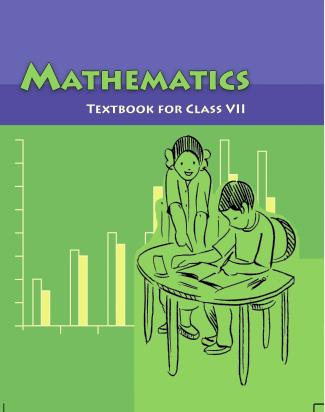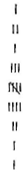1. /
2. CBSE
3. /
4. Class 07
5. /
6. Mathematics
7. /
8. NCERT Solutions for Class...

# NCERT Solutions for Class 7 Maths Exercise 3.1### myCBSEguide App

Download the app to get CBSE Sample Papers 2023-24, NCERT Solutions (Revised), Most Important Questions, Previous Year Question Bank, Mock Tests, and Detailed Notes.

NCERT solutions for Maths Data Handling## NCERT Solutions for Class 7 Maths Data Handling

###### Question 1.Find the range of heights of any ten students of your class.

 S. No. Name of students Height (in feet) 1 Gunjan 4.2 2 Aditi 4.5 3 Nikhil 5 4 Akhi 5.1 5 Riya 5.2 6 Akshat 5.3 7 Abhishek 5.1 8 Mayank 4.7 9 Rahul 4.9 10 Ayush 4.5

Range = Highest height – Lowest height

= 5.3 – 4.2

= 1.1 feet.

###### Question 2.Organize the following marks in a class assessment, in a tabular form:

4, 6, 7, 5, 3, 5, 4, 5, 2, 6, 2, 5, 1, 9, 6, 5, 8, 4, 6, 7.

(i)Which number is the highest?

(ii)Which number of the lowest?

(iii) What is the range of the lowest?

(iv) Find the arithmetic mean

 S. No. Marks Tally marks Frequency (No. of students) 1 11 2 2 2 3 3 1 4 4 3 5 5 5 6 6 4 7 7 2 8 8 1 9 9 1

(i)The highest number is 9.

(ii)The lowest number is 1.

(iii)The range of the data is 9 – 1 = 8

(iv)Arithmetic mean =

{tex}\frac{{4 + 6 + 7 + 5 + 3 + 5 + 4 + 5 + 2 + 6 + 2 + 5 + 1 + 9 + 6 + 5 + 8 + 4 + 6 + 7}}{{20}}{/tex}

= {tex}\frac{{100}}{{20}}{/tex} =5

NCERT Solutions for Class 7 Maths Exercise 3.1

###### Question 3.Find the mean of the first five whole numbers.

The first five whole numbers are 0, 1, 2, 3 and 4.

Therefore, Mean of first five whole numbers = {tex}\frac{{{\text{Sum of numbers}}}}{{{\text{Total number}}}}{/tex}

= {tex}\frac{{0 + 1 + 2 + 3 + 4}}{5}{/tex} = {tex}\frac{{10}}{5}{/tex} =2

Thus, the mean of first five whole numbers is 5.

NCERT Solutions for Class 7 Maths Exercise 3.1

###### Question 4.A cricketer scores the following runs in eight innings: 58, 76, 40, 35, 46, 45, 0, 100 Find the mean score.

Number of innings = 8

Mean of score = {tex}\frac{{{\text{Sum of scores}}}}{{{\text{Number of innings}}}}{/tex}

= {tex}\frac{{58 + 76 + 40 + 35 + 46 + 45 + 0 + 100}}{8}{/tex} = {tex}\frac{{400}}{8}{/tex} = 50

Thus, the mean score is 50.

NCERT Solutions for Class 7 Maths Exercise 3.1

###### Question 5.Following table shows the points of each player scored in four games:
 Player Game 1 Game 2 Game 3 Game 4 A 14 16 10 10 B 0 8 6 4 C 8 11 Did not play 13

(i) Find the mean to determine A’s average number of points scored per game.Now answer the following questions:

(ii) To find the mean number of points per game for C, would you divide the total points by 3 or 47? Why?

(iii) B played in all the four games. How would you find the mean?

(iv)Who is the best performer?

(i) Mean of player A = {tex}\frac{{{\text{Sum of scores by A}}}}{{{\text{No}}{\text{. of games played by A}}}}{/tex}

= {tex}\frac{{14 + 16 + 10 + 10}}{4} = \frac{{50}}{4}{/tex} = 12.5

(ii) We should divide the total points by 3 because player C played only three games.

(iii) Player B played in all the four games.

{tex}\therefore {/tex} Mean of player B = {tex}\frac{{{\text{Sum of scores by B}}}}{{{\text{No}}{\text{. of games played by B}}}}{/tex}

= {tex}\frac{{0 + 8 + 6 + 4}}{4} = \frac{{18}}{4}{/tex} = 4.5

(iv) To find the best performer, we should know the mean of all players.

Mena of player A = 12.5

Mean of player B = 4.5

Mean of player C = {tex}\frac{{8 + 11 + 13}}{3} = \frac{{32}}{3}{/tex} = 10.67

Therefore, on comparing means of all players, player A is the best performer.

NCERT Solutions for Class 7 Maths Exercise 3.1

###### Question 6.The marks (out of 100) obtained by a group of students in a science test are 85, 76, 90, 85, 39, 48, 56, 95, 81 and 75. Find the:

(i)The highest and the lowest marks obtained by the students.

(ii)Range of the marks obtained

(iii) Mean marks obtained by the group.

(i) Highest marks obtained by the student = 95

Lowest marks obtained by the student = 39

(ii) Range of marks = Highest marks – Lowest marks

= 95 – 39 = 56

(iii) Mean of obtained marks = {tex}\frac{{{\text{Sum of marks}}}}{{{\text{Total number of marks}}}}{/tex}

= {tex}\frac{{85 + 76 + 90 + 85 + 39 + 48 + 56 + 95 + 81 + 75}}{{10}}{/tex}

= {tex}\frac{{730}}{{10}}{/tex} = 73

Thus, mean marks obtained by the group of students is 73.

NCERT Solutions for Class 7 Maths Exercise 3.1

###### Question 7.The enrolment in a school during six consecutive years was as follows:

1555, 1670, 1750, 2013, 2540, 2820. Find the mean enrolment of the school for this period.

Mean enrolment = {tex}\frac{{{\text{Sum of numbers of enrolment}}}}{{{\text{Total number of enrolment}}}}{/tex}

= {tex}\frac{{1555 + 1670 + 1750 + 2013 + 2540 + 2820}}{6}{/tex}

= {tex}\frac{{12348}}{6}{/tex} = 2058

Thus, the mean enrolment of the school is 2,058.

NCERT Solutions for Class 7 Maths Exercise 3.1

###### Question 8.The rainfall (in mm) in a city on 7 days of a certain week was recorded as follows:
 Day Mon Tue Wed Thurs Fri Sat Sun Rainfall (in mm) 0 12.2 2.1 0 20.5 5.5 1

(i)Find the range of the rainfall in the above data.

(ii)Find the mean rainfall for the week.

(iii)On how many days was the rainfall less than the mean rainfall?

(i) The range of the rainfall = Highest rainfall – Lowest rainfall

= 20.5 – 0.0 = 20.5 mm

(ii) Main rainfall = {tex}\frac{{{\text{Sum of rainfall recorded}}}}{{{\text{Total number of days}}}}{/tex}

= {tex}\frac{{0.0 + 12.2 + 2.1 + 2.2 + 20.5 + 5.5 + 1.0}}{7}{/tex}

= {tex}\frac{{41.3}}{7}{/tex} = 5.9 mm

(iii) 5 days. i.e., Monday, Wednesday, Thursday, Saturday and Sunday rainfalls were less than the mean rainfall.

NCERT Solutions for Class 7 Maths Exercise 3.1

###### Question 9.The height of 10 girls were measured in cm and the results are as follows:

135, 150, 139, 128, 151, 132, 146, 149, 143, 141

(i)What is the height of the tallest girl?

(ii)What is the height of the shortest girl?

(iii)What is the range of data?

(iv)What is the mean height of the girls?

(v)How many girls have heights more than the mean height?

(i) The height of the tallest girl = 151 cm

(ii) The height of the shortest girl = 128 cm

(iii) The range of the data = Highest height – Lowest height

= 151 – 128 = 23 cm

(iv) The mean height = {tex}\frac{{{\text{Sum of heights of the girsl}}}}{{{\text{Total numebr of girls}}}}{/tex}

= {tex}\frac{{135 + 150 + 139 + 128 + 151 + 132 + 146 + 149 + 143 + 141}}{{10}}{/tex}

= {tex}\frac{{1414}}{{10}}{/tex} = 141.4 cm

(v) Five girls, i.e., 150, 151, 146, 149, 143 have heights (in cm) more than the mean height.

## NCERT Solutions for Class 7 Maths Exercise 3.1

NCERT Solutions Class 7 Maths PDF (Download) Free from myCBSEguide app and myCBSEguide website. Ncert solution class 7 Maths includes text book solutions from Class 7 Maths Book . NCERT Solutions for CBSE Class 7 Maths have total 15 chapters. 7 Maths NCERT Solutions in PDF for free Download on our website. Ncert Maths class 7 solutions PDF and Maths ncert class 7 PDF solutions with latest modifications and as per the latest CBSE syllabus are only available in myCBSEguide.

## CBSE app for Students

To download NCERT Solutions for Class 7 Maths, Social Science Computer Science, Home Science, Hindi English, Maths Science do check myCBSEguide app or website. myCBSEguide provides sample papers with solution, test papers for chapter-wise practice, NCERT solutions, NCERT Exemplar solutions, quick revision notes for ready reference, CBSE guess papers and CBSE important question papers. Sample Paper all are made available through the best app for CBSE students and myCBSEguide website.### Test Generator

Create question paper PDF and online tests with your own name & logo in minutes.### myCBSEguide

Question Bank, Mock Tests, Exam Papers, NCERT Solutions, Sample Papers, Notes

### 5 thoughts on “NCERT Solutions for Class 7 Maths Exercise 3.1”

1. Thanks brother or sister this help me much in my school home work

2. Hi mercy of the book of your life is good news

3. Wow

4. He is very good

5. Thanks bro or sis I got all sums perfectly thank you soo much?????????